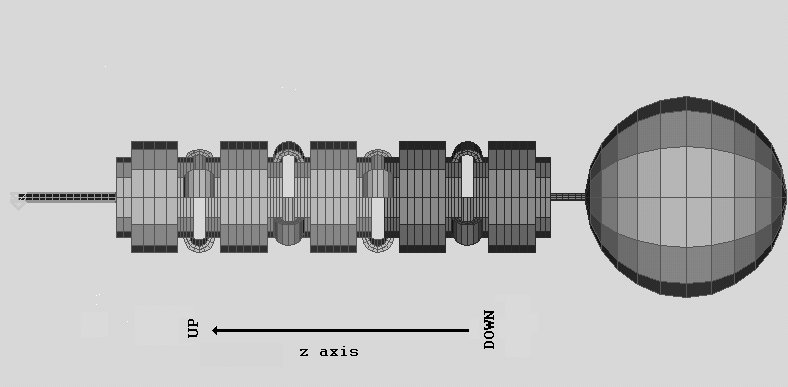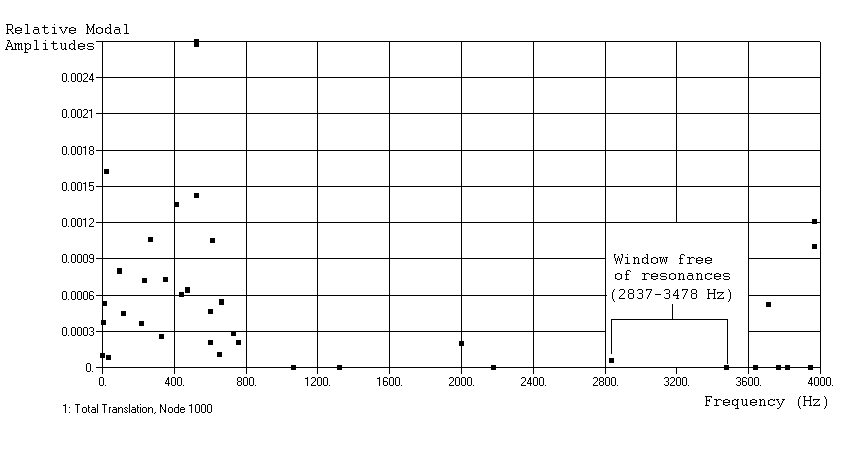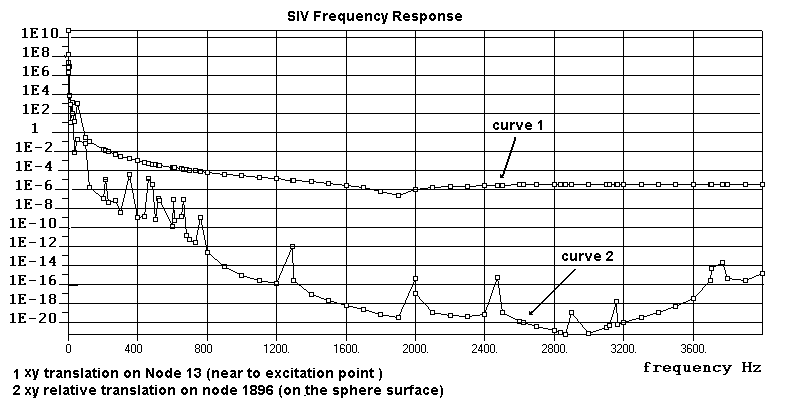## Gravity.uwa.edu.au

Vibration Isolation Support System for SCHENBERG Detector
J.L. Melo, W.F. Velloso Jr. and O. D. Aguiar
Instituto Nacional de Pesquisas Espaciais - INPE .
Avenida dos Astronautas, 1758
São José dos Campos, SP 12227-010, Brazil
e-mail: ze@das.inpe.br
Abstract: We designed a mechanical isolation system for a spherical resonant gravitational
wave detector we are building in Brazil. We have used the Finite Element Method to
perform the dynamical analysis. The system is a multiple stage passive pendulum formed by
cylinders joined by C springs and rods. Our results showed that the designed system could
allow a 280 dB attenuation factor in the bandwidth, from 3.1 kHz to 3.2 kHz, where the
SCHENBERG detector will be sensitive.
1. Introduction
The Brazilian Graviton Project Group is presently constructing the “Mario SCHENBERG Detector” - a 0,65m diameter spherical gravitational wave detector made of CuAl6%. We have designed the vibration isolationsystem for the SCHENBERG detector. The basic idea used in the system design was to construct amechanical multi-stage low pass filter capable of filtering all the mechanical vibration noises. The dynamicalbehavior of the system was numerically simulated using the Finite Element Method1 (FEM).
2 .The Isolation Support System Designed for SCHENBERG DETECTOR
With the SCHENBERG detector, we expect to obtain a spectral sensitivity of about 10-22m/(Hz)1/2, whichmeans that is necessary to attenuate the seismic noise by a factor of 10-10 , or -200 dB, at least, in the spectralrange of interest (3100Hz). So it is necessary to construct an isolation system capable of providing this levelof attenuation. The classic way to obtain such high attenuation is to use a series of spring-mass modules in amulti-stage set-up2. As is well known, this type of structure works as a low-pass filter and so provides anattenuation to mechanical noises. This kind of isolation system is normally used both in interferometers andresonant antennas3.
The figure 1 shows a schematics of the structure we are proposing: five cylinders joined together by six setsof C springs compose the system. The C springs connect the upper face of a cylinder to the bottom face of theimmediately above one. The use of C shaped springs in isolation systems for gravitational waves detectionwas first proposed by AURIGA group.
The basic design constraints we used were: 1) the cylinders must be small enough to have the first internalresonance at a frequency higher and far from the detection frequency; and 2) to choose the geometricalparameters of the springs (internal and external radius and widths) in such a way to adjust the elasticcharacteristics in order to obtain a spectral window, as large as possible, around the detection frequency,where there are not internal resonances.The top three cylinders and their C springs, as well as the resonant sphere itself, will be made of Cu-Al(6%)alloy (light gray in Figure 1). The two lower cylinders and tubes will be made by OFHC (Oxygen Free Highpurity Copper). At last we have the higher tube which will be Titanium made, since this material has higheryield stress values, allowing to use a tube with a smaller diameter; which decrease the heat flux betweenoutside and inside the detector.
Figure 1 - Finite Elements Model of the SCHENBERG isolation system with the resonant mass.
Our numerical FEM model also includes the resonant sphere. The simulation of both the resonant sphere andthe isolation system in the same model allows to analyze the frequency response directly in the surface of thesphere at the position where the transducers will be assembled.
3 . THE FINITE ELEMENT ANALYSIS: NUMERICAL SIMULATIONS
Using the above considerations we defined a first geometric set-up for the vibrational isolation system. Wehave numerically modeled the structure using a large number of finite elements in order to increase the FEMcalculation accuracy. We also conceived a geometrical configuration as symmetrical as possible (see Fig. 1)to simplify the numerical solutions.
As a first step of the analysis we used the FEM to calculate the stress of the structure, considering the totalweight (including, of course, the mass of the resonant sphere). It’s a design requirement that the stress on themodel must be from 10% to 20% of the yield stress, since the phonon production must be the least possible.
The OFHC yield stress is 3 x 109 dyn/cm2 and the Cu-Al6% yield stress is 1.3 x 109 dyn/cm2 . Of course, themaximum stress in the structure occurs in the C spring middle at the top of the system. In the proposed modelthis maximum stress value is 2.5 x 108 dyn/cm2, which corresponds to only 20% of the yield stress for theOFHC. In all other elements of the model, the stress was under this value, which means that the materials inall the structure are far enough from the non-linear region in way to prevent the occurrence of up-conversionphenomena by body stress relaxation.
In Figure 2 we show the calculated normal modes, using the Finite Element Method, for the proposedisolation system set-up, in a large spectral band (from 0 Hz to 4000Hz ) that includes the detectorcharacteristic frequency (3100Hz) . With this set-up we obtained a kind of spectral window, from 2837 Hz to3478 Hz, where the structure has no internal resonances. This 640 Hz window is large enough to prevent thenoise increasing by the internal modes resonances and so to allow us to expect a large attenuation factoraround the detection frequency.
Figure 2 - Vibration Isolation System Normal Modes Frequencies.
4- DISCUSSION : DYNAMICAL ANALISYS AND THE FREQUENCY RESPONSE CURVES
Even if the proposed structure is not directly comparable with a simple multi-stage uncoupled pendulum,since we must to consider also the internal high frequency resonances of the rigid springs, we can expect toachieve a good attenuation level at frequencies far enough from the first modes. It is possible to calculate thefrequency response solving numerically the dynamical equations of the structure, considering also a force ofexcitation applied to the structure.
The frequency response will be calculated by: P(w)} [− w M + K] Where M and K are respectively the matrices for the mass distribution and elastic constants and P(w) is anexcitation force ( acceleration ) applied to the structure. To perform our calculation, we have used a highacceleration amplitude signal, but we have imposed to P(w) a spectral dependence similar to the classical4seismic noise behavior given by αω-2, where α depends on the geographical coordinates on Earth surface.
It would be necessary to consider at least frequencies from 0Hz to 4000Hz, which means computing allamplitudes in the six degrees-of-freedom for each element of the isolation structure for each frequency step.
To solve this problem we used the direct method5 which calculates “exactly” the numerical response. TheFigure 3 shows the results of this calculation. The curve1 corresponds to the spectral response of the systemin a node located near to the excitation point at the top of the structure. The curve 2 represents the frequencyresponse in a node located at the surface of the resonant sphere. The transfer function to the system can beestimated comparing both curves. In curve 2 it is also possible to see the sphere resonances ( torsional – at2886 Hz - and radial quadrupolar at 3157 Hz ) since we have used a large excitation signal in ourcalculations. The difference between the curves corresponds to the attenuation in each frequency. In thedetection region (form 3100 Hz to 3200 Hz) we obtained about 280 dB which is sufficient to achieve thesensitivity level we are expecting to the SCHENBERG Detector.
Figure 3 - Attenuation produced on the structure by the vibration isolation system
ACKNOWLEDGMENTS
This work has been funded by grants from FAPESP (proc. n. 1996/2238-7 and 1997/14437-7) and CNPq
(proc. n. 300 746/95-4 (NV) ) . The experimental data of yield stress for the Cu-Al alloy were obtained in
collaboration with C. Frajuca at CEFET in Sao Paulo ).

1 Norrie D., and Kardestuncer H. - The Finite Element Handbook. 1987, MacGraw Hill, New York
2 Velloso Jr. W.F, Melo J.L. and Aguiar O.D. - AIP Conference Proc. 523,1999, p441
3 Velloso Jr. W.F, Melo J.L. and Aguiar O.D. - Review of Scientific Instruments, 2000 , Vol71, N6
4 Araya A. , Kawabe K., Sato T., Mio N. and Tsubono K., Rev . Sci. Instrum. 1993, 64, 5
5 Astley R.J. - Finite Elements in Solids and Structures, 1992, Chapman & Hall ed., London

Source: http://www.gravity.uwa.edu.au/amaldi/papers/Melo.pdf

### Protocol

Protocol STER onderzoek ST atus E pilepticus na R eanimatie A. Bouwes1, J.M. Binnekade1, J.H.T.M. Koelman2, A. Hijdra3, J. Horn11 Dept of Intensive Care AMC, 2 Dept of Clinical Neurophysiology AMC, 3 Dept of J. Horn, Intensive Care, Academic Medical Center, Meibergdreef 9, 1105 AZ, Inhoudsopgave Jaarlijks worden er in Nederland ongeveer 7500 patiënten opgenomen na een reanim

### Corel ventura - untitled.chp

Signs of Inequality: Constructing Disability in Antidepressant Drug Advertising Patricia Peppin* & Elaine Carty** Abstract Drug advertising constructs our understanding of disability. Drug ads use imageryto represent drugs, the disease conditions for which they are intended, the doctor-patient relationship, and the people with the condition. This imagery, understoodthrough semiotic